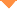首页 > 论文 > 激光与光电子学进展 > 56卷 > 12期(pp：122201--1)

Fast Convergence Stochastic Parallel Gradient Descent Algorithm

• 摘要• 论文信息• 参考文献• 被引情况• PDF全文Abstract

In this paper, based on deformable mirrors and the stochastic parallel-gradient-descent (SPGD) algorithm, an adaptive optics system (AOS) without wavefront detection is theoretically simulated. In order to improve the convergence speed of the AOS without reducing its accuracy, this paper optimizes the relationship between the amplitude of random perturbation and the gain coefficient in the SPGD algorithm. The experiment conducted in this study shows that the AOS has a parameter preference area, which is related to the initial distortion magnitude. Furthermore, the results of the theoretical verification and the comparison with that by the simulated annealing algorithm reveal that the convergence accuracy of the SPGD algorithm is 6.32% higher than that of the SA algorithm and the SPGD algorithm has a larger convergence speed.Dongting Hu, Wen Shen, Wenchao Ma, Xinyu Liu, Zhouping Su, Huaxin Zhu, Xiumei Zhang, Lizhi Que, Zhuowei Zhu, Yixin Zhang, Guoqing Chen, Lifa Hu. Fast Convergence Stochastic Parallel Gradient Descent Algorithm[J]. Laser & Optoelectronics Progress, 2019, 56(12): 122201